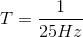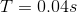# High School Physics : Understanding Amplitude and Period

## Example Questions

### Example Question #1 : Understanding Amplitude And Period

A wave has a frequency of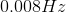. What is its period?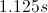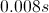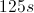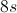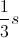Explanation:

The relationship between frequency and period is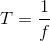Plug in our given value: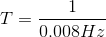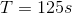### Example Question #32 : Waves

A wave has a period of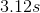. What is its frequency?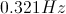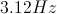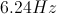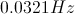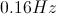Explanation:

The relationship between frequency and period is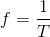Plug in our given value: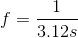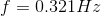### Example Question #33 : Waves

A certain type of radiation on the electromagnetic spectrum has a frequency of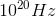. What is the period of this radiation?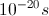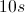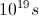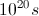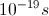Explanation:

The relationship between frequency and period is:We are given the frequency in the question. Using this value, we can solve for the period.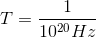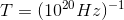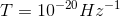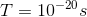### Example Question #661 : High School Physics

What is the frequency of a wave with a period of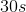?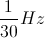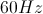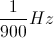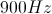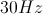Explanation:

The relationship between frequency and period is:Frequency is simply the reciprocal of period.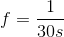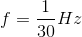### Example Question #2 : Understanding Amplitude And Period

A saxophone plays a note with a wavelength of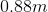. What is the period of the sound?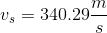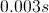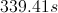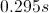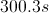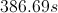Explanation:

The relationship between wavelength, frequency, and velocity is: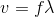Frequency is the inverse of period, allowing us to substitute into the velocity equation.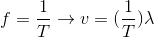We are given the speed of sound and the wavelength. Using these values, we can solve for the period.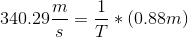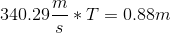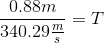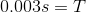### Example Question #1 : Understanding Amplitude And Period

A bird chirpstimes per second. What is the period of the chirping?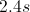There is insufficient information to solve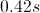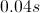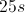Explanation:

The problem gives us a frequency in chirps per second. This is the same as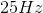as a "chirp" has no dimensional value.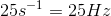Period is given by the reciprocal of frequency.Using our known frequency, we can solve for the period.